# Meeting 3. Gauss's Law.pptx

31 de Mar de 2023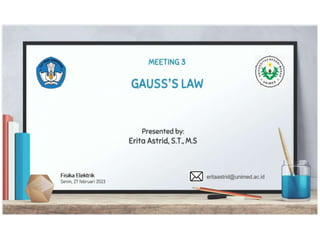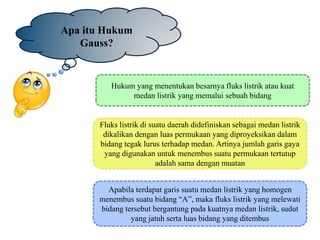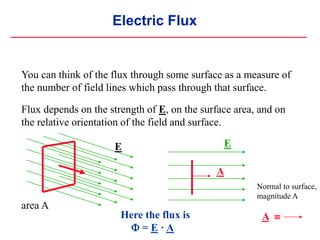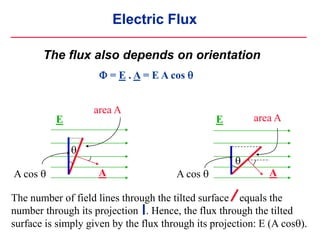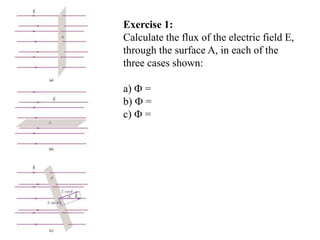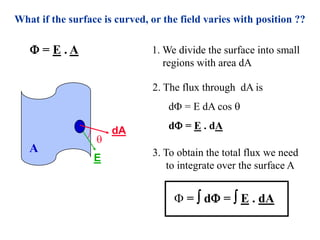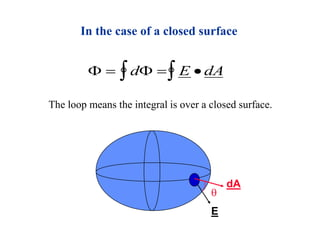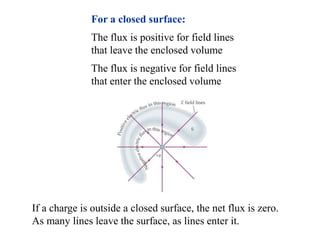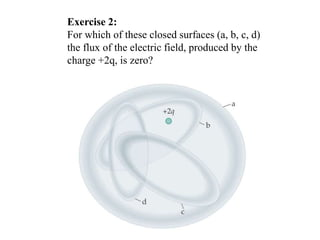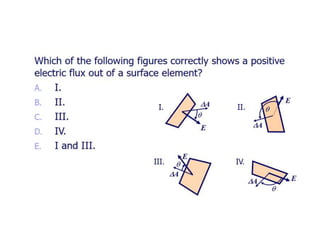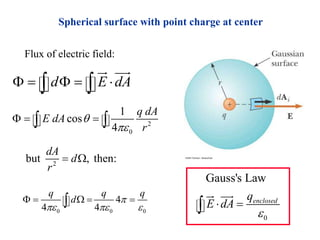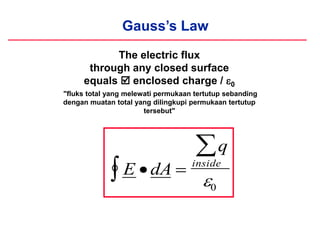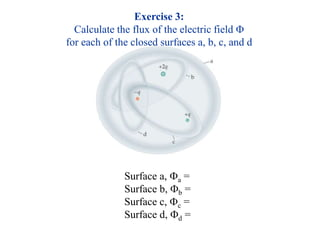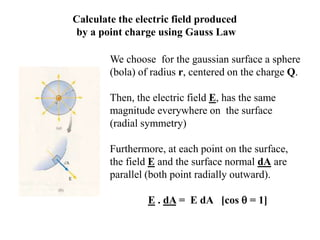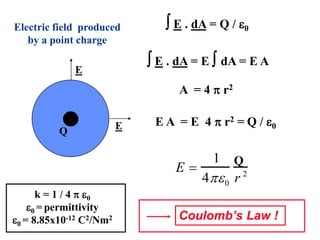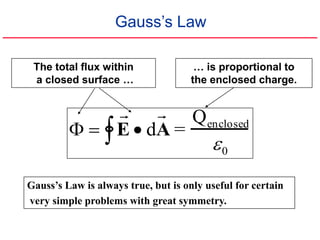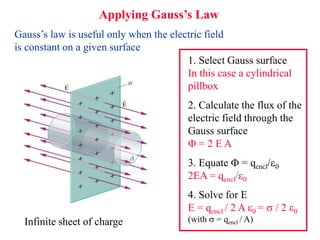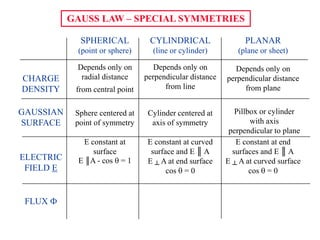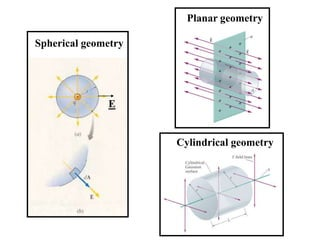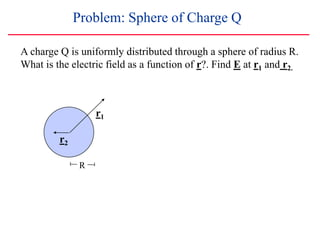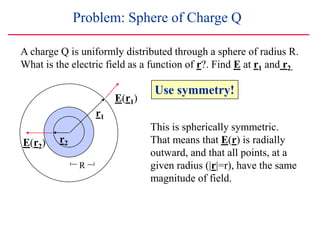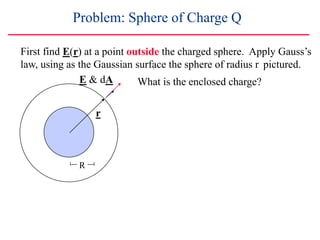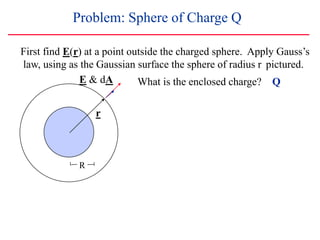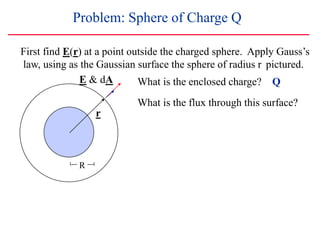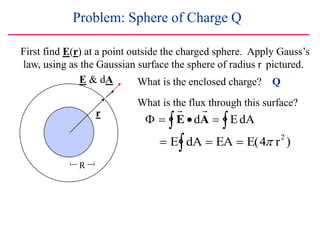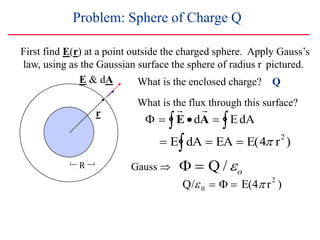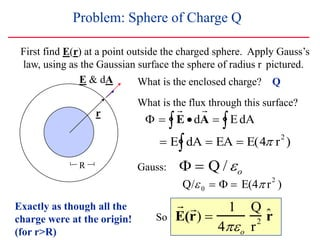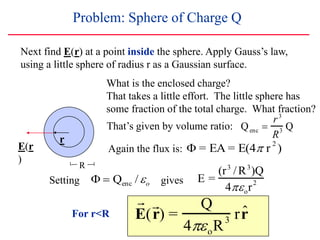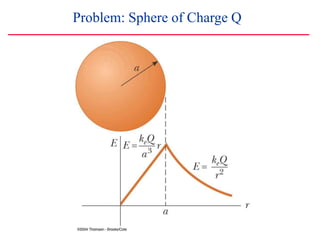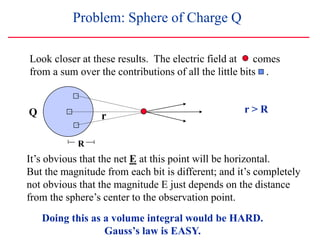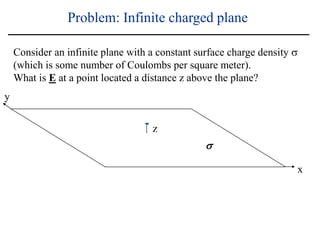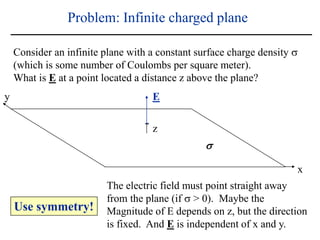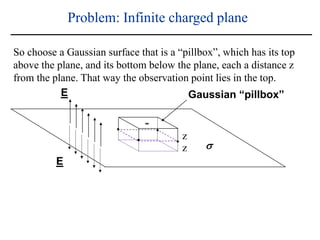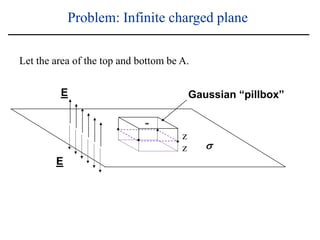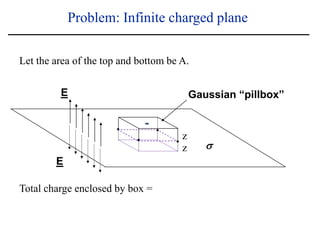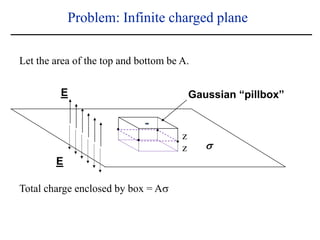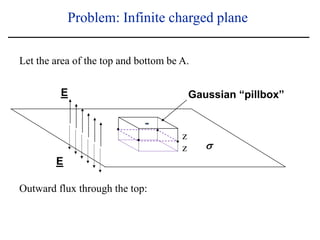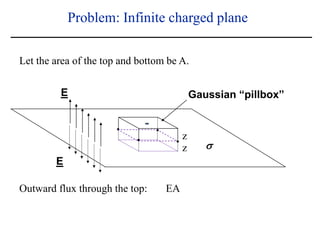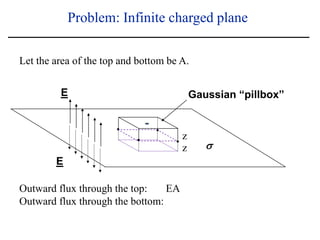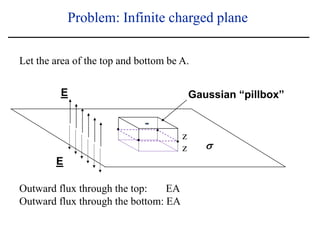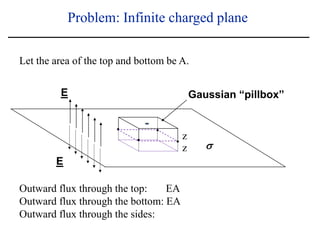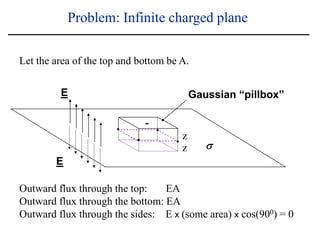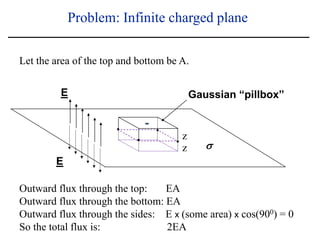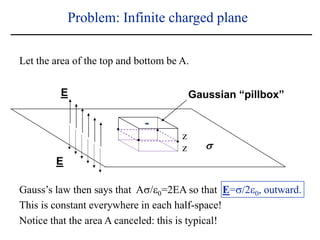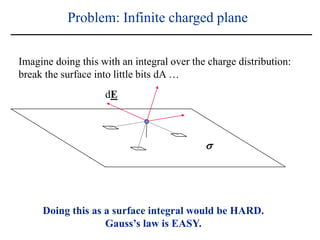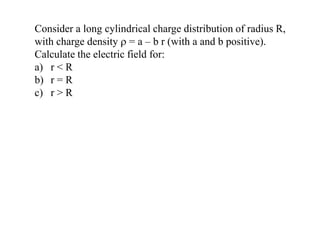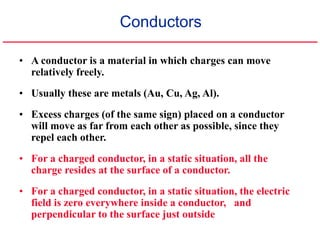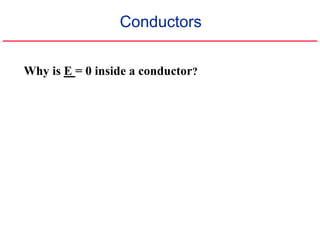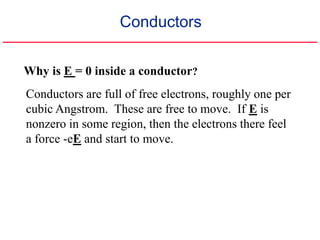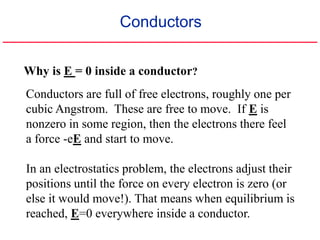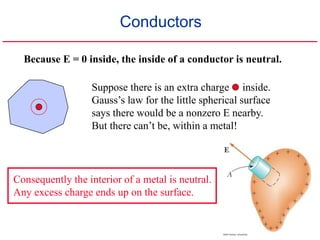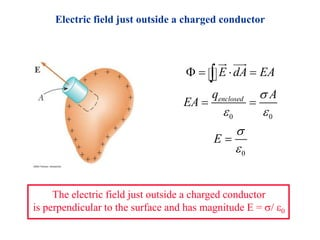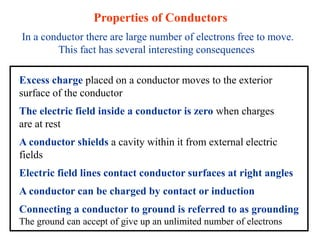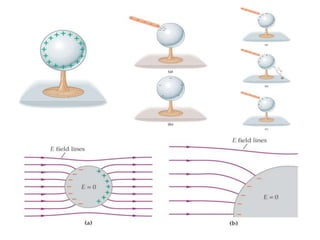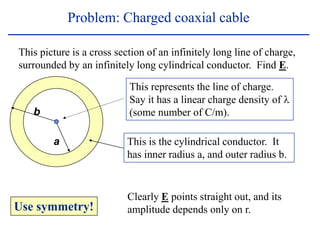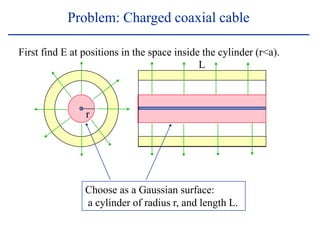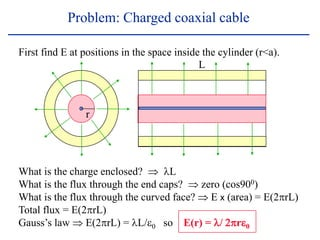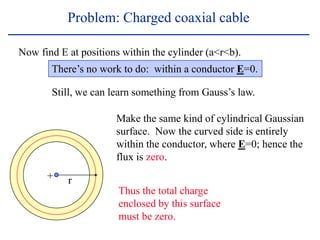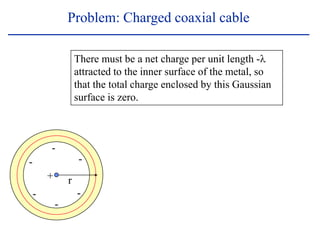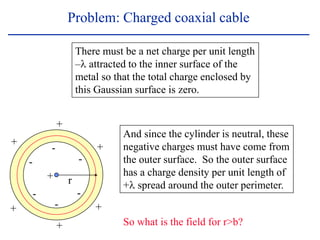1 de 63

### Meeting 3. Gauss's Law.pptx

1. Apa itu Hukum Gauss? Hukum yang menentukan besarnya fluks listrik atau kuat medan listrik yang memalui sebuah bidang Fluks listrik di suatu daerah didefiniskan sebagai medan listrik dikalikan dengan luas permukaan yang diproyeksikan dalam bidang tegak lurus terhadap medan. Artinya jumlah garis gaya yang digunakan untuk menembus suatu permukaan tertutup adalah sama dengan muatan Apabila terdapat garis suatu medan listrik yang homogen menembus suatu bidang “A”, maka fluks listrik yang melewati bidang tersebut bergantung pada kuatnya medan listrik, sudut yang jatuh serta luas bidang yang ditembus
2. Electric Flux We define the electric flux , of the electric field E, through the surface A, as:  = E . A Where: A is a vector normal to the surface (magnitude A, and direction normal to the surface).  is the angle between E and A area A E A  = E A cos ()
3. Electric Flux Here the flux is  = E · A You can think of the flux through some surface as a measure of the number of field lines which pass through that surface. Flux depends on the strength of E, on the surface area, and on the relative orientation of the field and surface. Normal to surface, magnitude A area A E A E A 
4. Electric Flux The flux also depends on orientation area A  A cos  The number of field lines through the tilted surface equals the number through its projection . Hence, the flux through the tilted surface is simply given by the flux through its projection: E (A cos).  = E . A = E A cos  area A  A cos  E E A A
5. Exercise 1: Calculate the flux of the electric field E, through the surface A, in each of the three cases shown: a)  = b)  = c)  =
6.  dA E What if the surface is curved, or the field varies with position ?? 1. We divide the surface into small regions with area dA 2. The flux through dA is d = E dA cos  d = E . dA 3. To obtain the total flux we need to integrate over the surface A A  =  d =  E . dA  = E . A
7. In the case of a closed surface          d E dA q inside 0 The loop means the integral is over a closed surface.  dA E
8. For a closed surface: The flux is positive for field lines that leave the enclosed volume The flux is negative for field lines that enter the enclosed volume If a charge is outside a closed surface, the net flux is zero. As many lines leave the surface, as lines enter it.
9. Exercise 2: For which of these closed surfaces (a, b, c, d) the flux of the electric field, produced by the charge +2q, is zero?
10. Spherical surface with point charge at center d E dA        Flux of electric field: 2 0 1 cos 4 q dA E dA r        2 but , then: dA d r   0 0 0 4 4 4 q q q d           0 Gauss's Law enclosed q E dA    
11. Gauss’s Law The electric flux through any closed surface equals  enclosed charge / 0 "fluks total yang melewati permukaan tertutup sebanding dengan muatan total yang dilingkupi permukaan tertutup tersebut"          d E dA q inside 0
12. Exercise 3: Calculate the flux of the electric field  for each of the closed surfaces a, b, c, and d Surface a, a = Surface b, b = Surface c, c = Surface d, d =
13. Calculate the electric field produced by a point charge using Gauss Law We choose for the gaussian surface a sphere (bola) of radius r, centered on the charge Q. Then, the electric field E, has the same magnitude everywhere on the surface (radial symmetry) Furthermore, at each point on the surface, the field E and the surface normal dA are parallel (both point radially outward). E . dA = E dA [cos  = 1]
14. E Q Coulomb’s Law ! E  1 40 q r 2  E . dA = Q / 0  E . dA = E  dA = E A A = 4  r2 E A = E 4  r2 = Q / 0 Electric field produced by a point charge E Q k = 1 / 4  0 0 = permittivity 0 = 8.85x10-12 C2/Nm2
15.   E  d  A = Qenclosed 0 Gauss’s Law The total flux within a closed surface … … is proportional to the enclosed charge. Gauss’s Law is always true, but is only useful for certain very simple problems with great symmetry.
16. Applying Gauss’s Law Gauss’s law is useful only when the electric field is constant on a given surface Infinite sheet of charge 1. Select Gauss surface In this case a cylindrical pillbox 2. Calculate the flux of the electric field through the Gauss surface  = 2 E A 3. Equate  = qencl/0 2EA = qencl/0 4. Solve for E E = qencl / 2 A 0 =  / 2 0 (with  = qencl / A)
17. GAUSS LAW – SPECIAL SYMMETRIES SPHERICAL (point or sphere) CYLINDRICAL (line or cylinder) PLANAR (plane or sheet) CHARGE DENSITY Depends only on radial distance from central point Depends only on perpendicular distance from line Depends only on perpendicular distance from plane GAUSSIAN SURFACE Sphere centered at point of symmetry Cylinder centered at axis of symmetry Pillbox or cylinder with axis perpendicular to plane ELECTRIC FIELD E E constant at surface E ║A - cos  = 1 E constant at curved surface and E ║ A E ┴ A at end surface cos  = 0 E constant at end surfaces and E ║ A E ┴ A at curved surface cos  = 0 FLUX 
18. Cylindrical geometry Planar geometry Spherical geometry E
19. A charge Q is uniformly distributed through a sphere of radius R. What is the electric field as a function of r?. Find E at r1 and r2. Problem: Sphere of Charge Q r2 r1 R
20. A charge Q is uniformly distributed through a sphere of radius R. What is the electric field as a function of r?. Find E at r1 and r2. Problem: Sphere of Charge Q Use symmetry! This is spherically symmetric. That means that E(r) is radially outward, and that all points, at a given radius (|r|=r), have the same magnitude of field. r2 r1 R E(r1) E(r2)
21. Problem: Sphere of Charge Q E & dA r R What is the enclosed charge? First find E(r) at a point outside the charged sphere. Apply Gauss’s law, using as the Gaussian surface the sphere of radius r pictured.
22. Problem: Sphere of Charge Q r R E & dA What is the enclosed charge? Q First find E(r) at a point outside the charged sphere. Apply Gauss’s law, using as the Gaussian surface the sphere of radius r pictured.
23. Problem: Sphere of Charge Q r R E & dA What is the enclosed charge? Q What is the flux through this surface? First find E(r) at a point outside the charged sphere. Apply Gauss’s law, using as the Gaussian surface the sphere of radius r pictured.
24. Problem: Sphere of Charge Q r R E & dA What is the enclosed charge? Q What is the flux through this surface?   E d  A  EdA   E dA   EA  E(4 r2 ) First find E(r) at a point outside the charged sphere. Apply Gauss’s law, using as the Gaussian surface the sphere of radius r pictured.
25. Problem: Sphere of Charge Q r R E & dA What is the enclosed charge? Q What is the flux through this surface?   E d  A  EdA   E dA   EA  E(4 r2 ) Gauss    Q /o Q/0    E(4 r 2 ) First find E(r) at a point outside the charged sphere. Apply Gauss’s law, using as the Gaussian surface the sphere of radius r pictured.
26. Problem: Sphere of Charge Q r R E & dA What is the enclosed charge? Q What is the flux through this surface?   E d  A  EdA   E dA   EA  E(4 r2 ) Gauss:   Q /o So E(r)  1 4o Q r 2 ˆ r Exactly as though all the charge were at the origin! (for r>R) Q/0    E(4 r 2 ) First find E(r) at a point outside the charged sphere. Apply Gauss’s law, using as the Gaussian surface the sphere of radius r pictured.
27. Problem: Sphere of Charge Q R r E(r ) Next find E(r) at a point inside the sphere. Apply Gauss’s law, using a little sphere of radius r as a Gaussian surface. What is the enclosed charge? That takes a little effort. The little sphere has some fraction of the total charge. What fraction? That’s given by volume ratio: Qenc  r3 R 3 Q Again the flux is:  = EA = E(4 r 2 ) Setting   Qenc /o gives E = (r3 /R3 )Q 4or 2 E(r) = Q 4oR3 r ˆ r For r<R
28. Problem: Sphere of Charge Q
29. Problem: Sphere of Charge Q Look closer at these results. The electric field at comes from a sum over the contributions of all the little bits . It’s obvious that the net E at this point will be horizontal. But the magnitude from each bit is different; and it’s completely not obvious that the magnitude E just depends on the distance from the sphere’s center to the observation point. Doing this as a volume integral would be HARD. Gauss’s law is EASY. R Q r r > R
30.  Problem: Infinite charged plane Consider an infinite plane with a constant surface charge density  (which is some number of Coulombs per square meter). What is E at a point located a distance z above the plane? x y z
31.  Problem: Infinite charged plane Consider an infinite plane with a constant surface charge density  (which is some number of Coulombs per square meter). What is E at a point located a distance z above the plane? x y z Use symmetry! The electric field must point straight away from the plane (if  > 0). Maybe the Magnitude of E depends on z, but the direction is fixed. And E is independent of x and y. E
32. E E Gaussian “pillbox”  Problem: Infinite charged plane So choose a Gaussian surface that is a “pillbox”, which has its top above the plane, and its bottom below the plane, each a distance z from the plane. That way the observation point lies in the top. z z
33. E E Gaussian “pillbox”  Problem: Infinite charged plane z z Let the area of the top and bottom be A.
34. E E Gaussian “pillbox”  Problem: Infinite charged plane z z Let the area of the top and bottom be A. Total charge enclosed by box =
35. E E Gaussian “pillbox”  Problem: Infinite charged plane z z Let the area of the top and bottom be A. Total charge enclosed by box = A
36. E E Gaussian “pillbox”  Problem: Infinite charged plane z z Let the area of the top and bottom be A. Outward flux through the top:
37. E E Gaussian “pillbox”  Problem: Infinite charged plane z z Let the area of the top and bottom be A. Outward flux through the top: EA
38. E E Gaussian “pillbox”  Problem: Infinite charged plane z z Let the area of the top and bottom be A. Outward flux through the top: EA Outward flux through the bottom:
39. E E Gaussian “pillbox”  Problem: Infinite charged plane z z Let the area of the top and bottom be A. Outward flux through the top: EA Outward flux through the bottom: EA
40. E E Gaussian “pillbox”  Problem: Infinite charged plane z z Let the area of the top and bottom be A. Outward flux through the top: EA Outward flux through the bottom: EA Outward flux through the sides:
41. E E Gaussian “pillbox”  Problem: Infinite charged plane z z Let the area of the top and bottom be A. Outward flux through the top: EA Outward flux through the bottom: EA Outward flux through the sides: E x (some area) x cos(900) = 0
42. E E Gaussian “pillbox”  Problem: Infinite charged plane z z Outward flux through the top: EA Outward flux through the bottom: EA Outward flux through the sides: E x (some area) x cos(900) = 0 So the total flux is: 2EA Let the area of the top and bottom be A.
43. E E Gaussian “pillbox”  Problem: Infinite charged plane z z Gauss’s law then says that A/0=2EA so that E=/20, outward. This is constant everywhere in each half-space! Let the area of the top and bottom be A. Notice that the area A canceled: this is typical!
44. Problem: Infinite charged plane Imagine doing this with an integral over the charge distribution: break the surface into little bits dA …  dE Doing this as a surface integral would be HARD. Gauss’s law is EASY.
45. Consider a long cylindrical charge distribution of radius R, with charge density  = a – b r (with a and b positive). Calculate the electric field for: a) r < R b) r = R c) r > R
46. • A conductor is a material in which charges can move relatively freely. • Usually these are metals (Au, Cu, Ag, Al). • Excess charges (of the same sign) placed on a conductor will move as far from each other as possible, since they repel each other. • For a charged conductor, in a static situation, all the charge resides at the surface of a conductor. • For a charged conductor, in a static situation, the electric field is zero everywhere inside a conductor, and perpendicular to the surface just outside Conductors
47. Conductors Why is E = 0 inside a conductor?
48. Conductors Why is E = 0 inside a conductor? Conductors are full of free electrons, roughly one per cubic Angstrom. These are free to move. If E is nonzero in some region, then the electrons there feel a force -eE and start to move.
49. Conductors Why is E = 0 inside a conductor? Conductors are full of free electrons, roughly one per cubic Angstrom. These are free to move. If E is nonzero in some region, then the electrons there feel a force -eE and start to move. In an electrostatics problem, the electrons adjust their positions until the force on every electron is zero (or else it would move!). That means when equilibrium is reached, E=0 everywhere inside a conductor.
50. Conductors Because E = 0 inside, the inside of a conductor is neutral. Suppose there is an extra charge inside. Gauss’s law for the little spherical surface says there would be a nonzero E nearby. But there can’t be, within a metal! Consequently the interior of a metal is neutral. Any excess charge ends up on the surface.
51. Electric field just outside a charged conductor 0 0 0 enclosed E dA EA q A EA E              The electric field just outside a charged conductor is perpendicular to the surface and has magnitude E = / 0
52. Properties of Conductors In a conductor there are large number of electrons free to move. This fact has several interesting consequences Excess charge placed on a conductor moves to the exterior surface of the conductor The electric field inside a conductor is zero when charges are at rest A conductor shields a cavity within it from external electric fields Electric field lines contact conductor surfaces at right angles A conductor can be charged by contact or induction Connecting a conductor to ground is referred to as grounding The ground can accept of give up an unlimited number of electrons
53. a b Problem: Charged coaxial cable This picture is a cross section of an infinitely long line of charge, surrounded by an infinitely long cylindrical conductor. Find E. This represents the line of charge. Say it has a linear charge density of l (some number of C/m). This is the cylindrical conductor. It has inner radius a, and outer radius b. Use symmetry! Clearly E points straight out, and its amplitude depends only on r.
54. Problem: Charged coaxial cable First find E at positions in the space inside the cylinder (r<a). Choose as a Gaussian surface: a cylinder of radius r, and length L. r L
55. What is the charge enclosed?  lL What is the flux through the end caps?  zero (cos900) What is the flux through the curved face?  E x (area) = E(2rL) Total flux = E(2rL) Gauss’s law  E(2rL) = lL/0 so E(r) = l/ 2r0 Problem: Charged coaxial cable First find E at positions in the space inside the cylinder (r<a). r L
56. Problem: Charged coaxial cable Now find E at positions within the cylinder (a<r<b). Make the same kind of cylindrical Gaussian surface. Now the curved side is entirely within the conductor, where E=0; hence the flux is zero. There’s no work to do: within a conductor E=0. Still, we can learn something from Gauss’s law. r + Thus the total charge enclosed by this surface must be zero.
57. Problem: Charged coaxial cable There must be a net charge per unit length -l attracted to the inner surface of the metal, so that the total charge enclosed by this Gaussian surface is zero. r + - - - - - -
58. Problem: Charged coaxial cable + + r + - - - - - - + + + + There must be a net charge per unit length –l attracted to the inner surface of the metal so that the total charge enclosed by this Gaussian surface is zero. And since the cylinder is neutral, these negative charges must have come from the outer surface. So the outer surface has a charge density per unit length of +l spread around the outer perimeter. So what is the field for r>b?